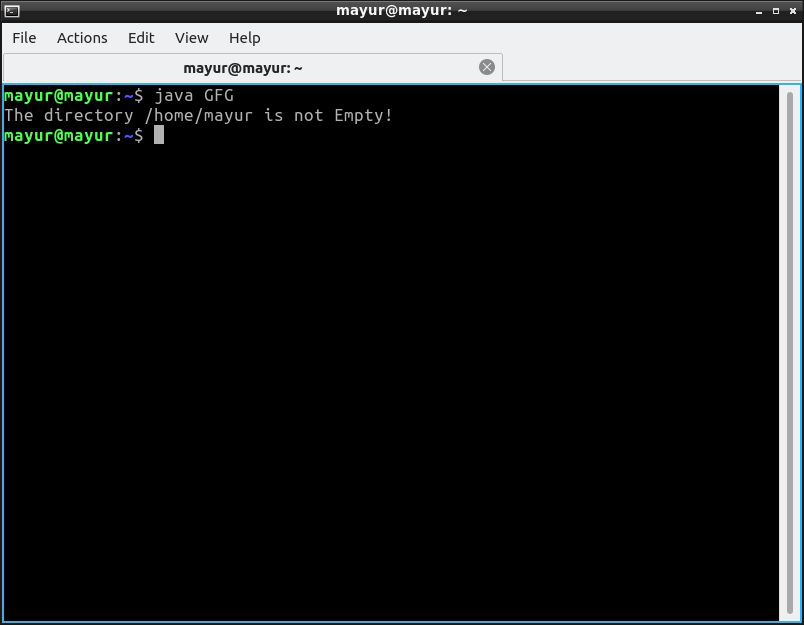GFG App
Open AppBrowser
Continue

# Java Program to Check if a Directory is Empty or Not

The list() method defined in class java.io.File which is used to obtain the list of files and folders(directories) present in the specified directory defined by its pathname. The list of files is stored in an array of string. If the length of an array is greater than 0, then the specified directory is not empty, else it is empty.

Method 1: Use of list() method

• Suppose there is a directory present in the path /home/user/folder and it has three text files.
• Create an array and store the name of files using the list() method.
• Calculate the length of a string array
• Print result

See the below program for the above approach.

## Java

 `// Java Program to check if  ` `// a directory is empty or not ` ` `  `import` `java.io.File; ` ` `  `class` `GFG { ` `   `  `    ``public` `static` `void` `main(String[] args) ` `    ``{ ` `        ``// mention the directory path ` `        ``File directory = ``new` `File(``"/home/mayur"``); ` ` `  `        ``if` `(directory.isDirectory()) { ` `           `  `            ``// creating a String Array ` `            ``// store name of files ` `            ``String arr[] = directory.list(); ` ` `  `            ``// check if length is greater than 0 or not ` `            ``if` `(arr.length > ``0``) { ` `                ``System.out.println(``"The directory "` `                                   ``+ directory.getPath() ` `                                   ``+ ``" is not Empty!"``); ` `            ``} ` `            ``else` `{ ` `                ``System.out.println(``"The directory "` `                                   ``+ directory.getPath() ` `                                   ``+ ``" is Empty!"``); ` `            ``} ` `        ``} ` `    ``} ` `}`

Output:Method 2: Use of DirectoryStream

Java 7 onwards, the Files.newDirectoryStream method was introduced that returns a DirectoryStream<Path> to iterate over all the entries in the directory.

• Suppose there is a directory present in the path /home/user/folder and it has three text files.
• Create a boolean function that checks if the directory is empty or not
• If a given path is a file then throw an exception
• If a given directory has files, return false else true.
• Print the result

See the below program for the above approach.

## Java

 `// Java Program to check if a ` `// directory is empty or not ` ` `  `import` `java.io.File; ` `import` `java.io.IOException; ` `import` `java.nio.file.DirectoryStream; ` `import` `java.nio.file.Files; ` `import` `java.nio.file.Path; ` ` `  `public` `class` `GFG { ` `   `  `    ``// boolean function which ` `    ``// return true if directory is ` `    ``// empty else return false ` `    ``public` `static` `boolean` `isEmptyDirectory(File directory) ` `        ``throws` `IOException ` `    ``{ ` `        ``// check if given path is a directory ` `        ``if` `(directory.exists()) { ` `            ``if` `(!directory.isDirectory()) { ` ` `  `                ``// throw exception if given path is a ` `                ``// file ` `                ``throw` `new` `IllegalArgumentException( ` `                    ``"Expected directory, but was file: "` `                    ``+ directory); ` `            ``} ` `            ``else` `{ ` `                ``// create a stream and check for files ` `                ``try` `(DirectoryStream directoryStream ` `                     ``= Files.newDirectoryStream( ` `                         ``directory.toPath())) { ` `                    ``// return false if there is a file ` `                    ``return` `!directoryStream.iterator() ` `                                ``.hasNext(); ` `                ``} ` `            ``} ` `        ``} ` `        ``// return true if no file is present ` `        ``return` `true``; ` `    ``} ` ` `  `    ``public` `static` `void` `main(String[] args) ` `        ``throws` `IOException ` `    ``{ ` `        ``// enter path of directory ` `        ``File directory = ``new` `File(``"/home/mayur"``); ` ` `  `        ``// call isEmptyDirectory Function ` `        ``if` `(isEmptyDirectory(directory)) { ` `            ``System.out.println(``"The directory "` `                               ``+ directory.getPath() ` `                               ``+ ``" is Empty!"``); ` `        ``} ` `        ``else` `{ ` `            ``System.out.println(``"The directory "` `                               ``+ directory.getPath() ` `                               ``+ ``" is Not Empty!"``); ` `        ``} ` `    ``} ` `}`

Output:My Personal Notes arrow_drop_up